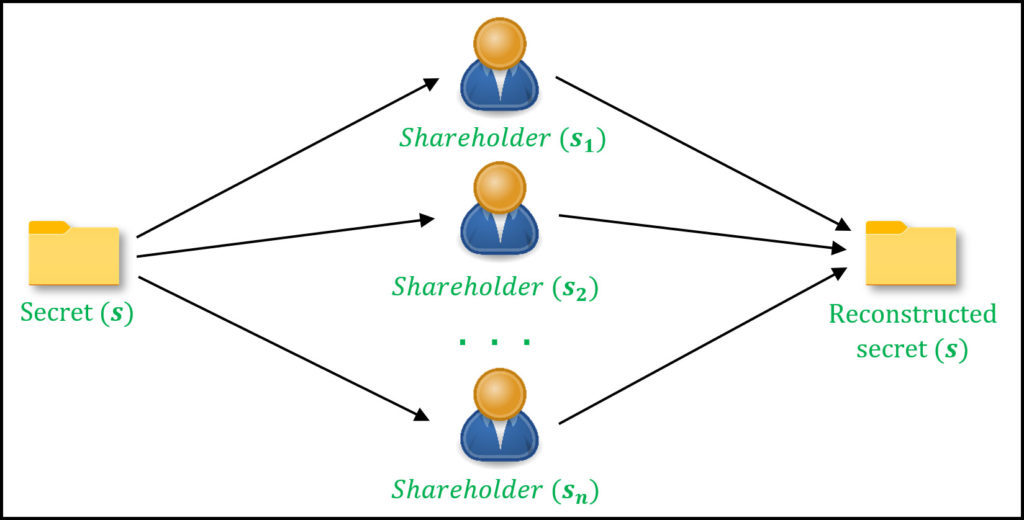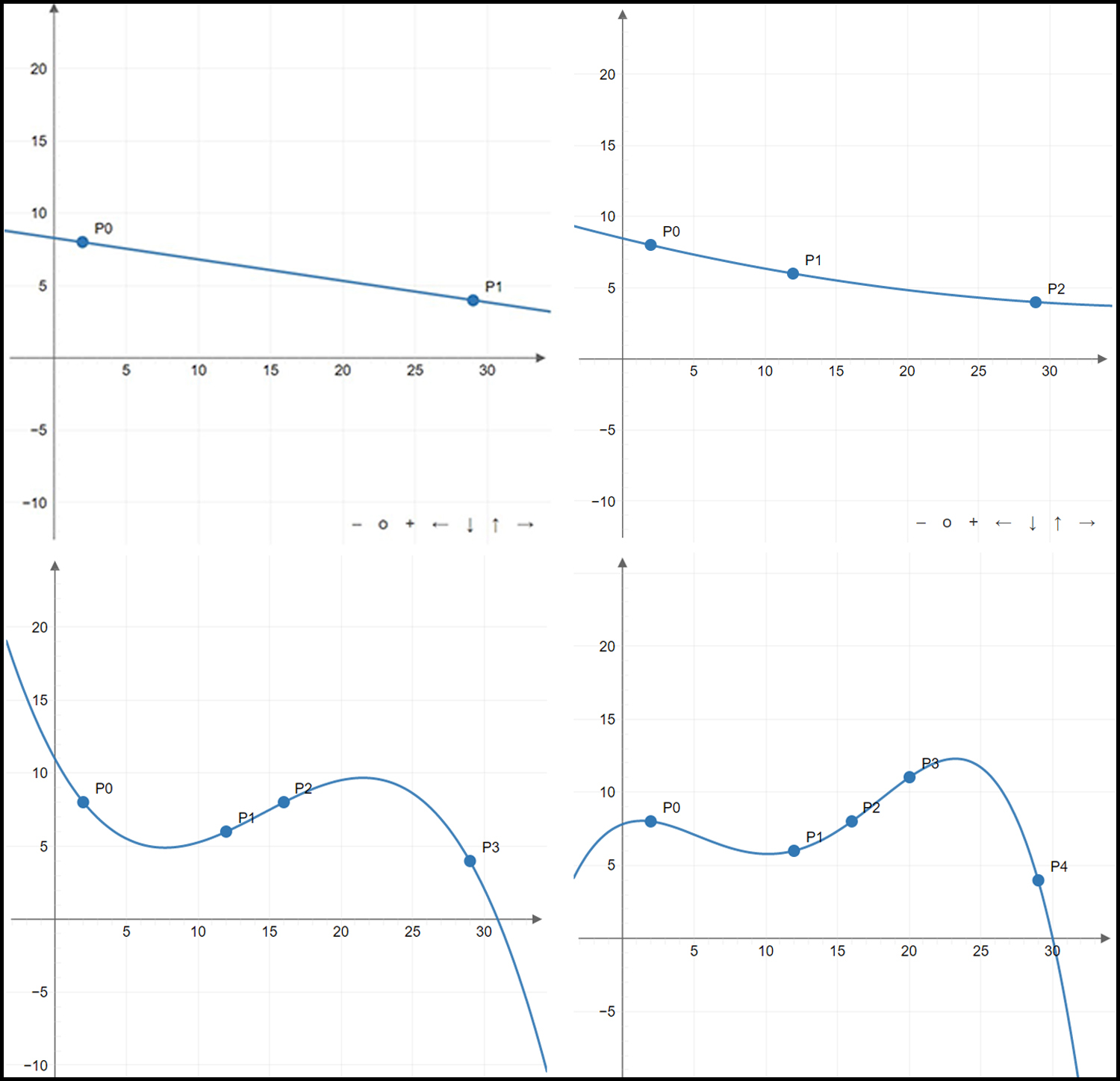# Implementing Shamir’s Secret Sharing Scheme in Python

Secret Sharing schemes are used in the distribution of a secret value among multiple participants (shareholders) by dividing the secret into fragments (shares). This is done in a manner that prevents a single shareholder from having complete knowledge of the original secret. The only way to retrieve the original secret is to combine the shares, distributed among the participants. Hence, the control of the secret is distributed. These schemes are examples of Threshold Cryptosystems, which involve the division of secrets among multiple parties such that several parties (more than some threshold number) must cooperate to reconstruct the secret.Fig 1: Depiction of Secret Sharing between n participants

In general, a secret may be split into n shares (for n shareholders), out of which, a minimum of t, (t < n) shares are required for successful reconstruction. Such a scheme is referred to as a `(t, n) sharing-scheme`. From the n participants, any subset of shareholders, of size greater or equal to t, can regenerate the secret.

## Shamir Secret Sharing

Shamir Secret Sharing(SSS) is one of the most popular implementations of a secret sharing scheme created by Adi Shamir, a famous Israeli cryptographer, who also contributed to the invention of RSA algorithm. SSS allows the secret to be divided into an arbitrary number of shares and allows an arbitrary threshold (as long as it is less than the total participants). SSS is based on the mathematical concept of polynomial interpolation which states A polynomial of degree `t-1` can be reconstructed from the knowledge of `t` or more points, known to be lying on the curve.

For instance, to reconstruct a curve of degree 1 (a straight line), we require at least 2 points that lie on the line. Conversely, it is mathematically infeasible to reconstruct a curve if the number of unique points available is less than (degree-of-curve + 1).

## Motivation behind SSS

The concept of polynomial interpolation can be applied to produce a secret sharing scheme by embedding the secret into the polynomial. A general polynomial of degree p can be expressed as follows:In the expression of`P(x)`, the values`a_{1}, a_{2}, a_{3}, ..., a_{n}` represent the coefficients of the polynomial. Thus, construction of a polynomial requires the selection of these coefficients.

Once the polynomial is generated, we can essentially represent the curve by just p+1 points that lie on the curve. This follows from the polynomial interpolation principle. For example, a curve of degree 4 can be reconstructed if we have access to atleast 5 unique points lying on it. To do this, we can run Lagrange’s interpolation or any other similar interpolation mechanism.Fig 2: Example of using Polynomial Interpolation to reconstruct a curve

Consequently, if we conceal the secret value into such a polynomial and use various points on the curve as shares, we arrive at a secret sharing scheme. More precisely, to establish a (t, n) secret sharing scheme, we can construct a polynomial of degree t-1 and pick n points on the curve as shares such that the polynomial will only be regenerated if t or more shares are pooled. The secret value (s) is concealed in the constant term (coefficient of 0 degree term) of the polynomial which can only be reconstructed after the successful reconstruction of the curve.

## Algorithm used

Shamir uses the polynomial interpolation principle to perform threshold sharing in the following two phases:
Phase I: Generation of Shares
This phase involves the setup of the system as well as the generation of the shares.

1. Decide the values for the number of participants (n) and the threshold (t) to secure some secret value (s)
2. Construct a random polynomial, P(x), with degree t-1 by choosing random coefficients of the polynomial. Set the constant term in the polynomial (coefficient of zero degree term) to be equal to the secret value s
3. To generate the n shares, randomly pick n points lying on the polynomial P(x)
4. Distribute the picked coordinates in the previous step among the participants. These act as the shares in the system

Phase II: Reconstruction of Secret
For reconstruction of the secret, a minimum of t participants are required to pool their shares.

1. Collect t or more shares
2. Use an interpolation algorithm to reconstruct the polynomial, P'(x), from the shares. Lagrange’s Interpolation is an example of such an algorithm
3. Determine the value of the reconstructed polynomial for x = 0, i.e. calculate P'(0). This value reveals the constant term of the polynomial which happens to be the original secret. Thus, the secret is reconstructed

Below is the implementation.

 `#Implementation of Shamir Secret Sharing Scheme ` `  `  `import` `random ` `from` `math ``import` `ceil ` `from` `decimal ``import` `*` `  `  `     `  `global` `field_size ` `field_size ``=` `10``*``*``5` `  `  `def` `reconstructSecret(shares): ` `     `  `    ``# Combines shares using  ` `    ``# Lagranges interpolation.  ` `    ``# Shares is an array of shares ` `    ``# being combined ` `    ``sums, prod_arr ``=` `0``, [] ` `     `  `    ``for` `j ``in` `range``(``len``(shares)): ` `        ``xj, yj ``=` `shares[j][``0``],shares[j][``1``] ` `        ``prod ``=` `Decimal(``1``) ` `         `  `        ``for` `i ``in` `range``(``len``(shares)): ` `            ``xi ``=` `shares[i][``0``] ` `            ``if` `i !``=` `j: prod ``*``=` `Decimal(Decimal(xi)``/``(xi``-``xj)) ` `                 `  `        ``prod ``*``=` `yj ` `        ``sums ``+``=` `Decimal(prod) ` `         `  `    ``return` `int``(``round``(Decimal(sums),``0``)) ` `  `  `def` `polynom(x,coeff): ` `     `  `    ``# Evaluates a polynomial in x  ` `    ``# with coeff being the coefficient ` `    ``# list ` `    ``return` `sum``([x``*``*``(``len``(coeff)``-``i``-``1``) ``*` `coeff[i] ``for` `i ``in` `range``(``len``(coeff))]) ` `  `  `def` `coeff(t,secret): ` `     `  `    ``# Randomly generate a coefficient  ` `    ``# array for a polynomial with ` `    ``# degree t-1 whose constant = secret''' ` `    ``coeff ``=` `[random.randrange(``0``, field_size) ``for` `_ ``in` `range``(t``-``1``)] ` `    ``coeff.append(secret) ` `     `  `    ``return` `coeff ` `  `  `def` `generateShares(n,m,secret): ` `     `  `    ``# Split secret using SSS into ` `    ``# n shares with threshold m ` `    ``cfs ``=` `coeff(m,secret) ` `    ``shares ``=` `[] ` `     `  `    ``for` `i ``in` `range``(``1``,n``+``1``): ` `        ``r ``=` `random.randrange(``1``, field_size) ` `        ``shares.append([r, polynom(r,cfs)]) ` `     `  `    ``return` `shares ` ` `  ` `  `# Driver code  ` `if` `__name__ ``=``=` `'__main__'``: ` `     `  `    ``# (3,5) sharing scheme ` `    ``t,n ``=` `3``, ``5` `    ``secret ``=` `1234` `    ``print``(``'Original Secret:'``, secret) ` `  `  `    ``# Phase I: Generation of shares ` `    ``shares ``=` `generateShares(n, t, secret) ` `    ``print``(``'\nShares:'``, ``*``shares) ` `  `  `    ``# Phase II: Secret Reconstruction ` `    ``# Picking t shares randomly for ` `    ``# reconstruction ` `    ``pool ``=` `random.sample(shares, t) ` `    ``print``(``'\nCombining shares:'``, ``*``pool) ` `    ``print``(``"Reconstructed secret:"``, reconstructSecret(pool)) `

Output:

Original Secret: 1234

Shares: [35592, 44789055659410] [71440, 180446310792274] [49416, 86337840016786] [2644, 247195070194] [33833, 40471410959526]

Combining shares: [33833, 40471410959526] [35592, 44789055659410] [2644, 247195070194]
Reconstructed secret: 1234

### Practical Applications

Secret sharing schemes are widely used in cryptosystems where trust is required to be distributed instead of centralized. Prominent examples of real world scenarios where secret sharing is used include:

• Threshold Based Signatures for Bitcoin
• Secure Multi-Party Computation
• Private Machine Learning with Multi Party Computation

My Personal Notes arrow_drop_upCheck out this Author's contributed articles.

If you like GeeksforGeeks and would like to contribute, you can also write an article using contribute.geeksforgeeks.org or mail your article to contribute@geeksforgeeks.org. See your article appearing on the GeeksforGeeks main page and help other Geeks.

Please Improve this article if you find anything incorrect by clicking on the "Improve Article" button below.

Article Tags :

3

Please write to us at contribute@geeksforgeeks.org to report any issue with the above content.